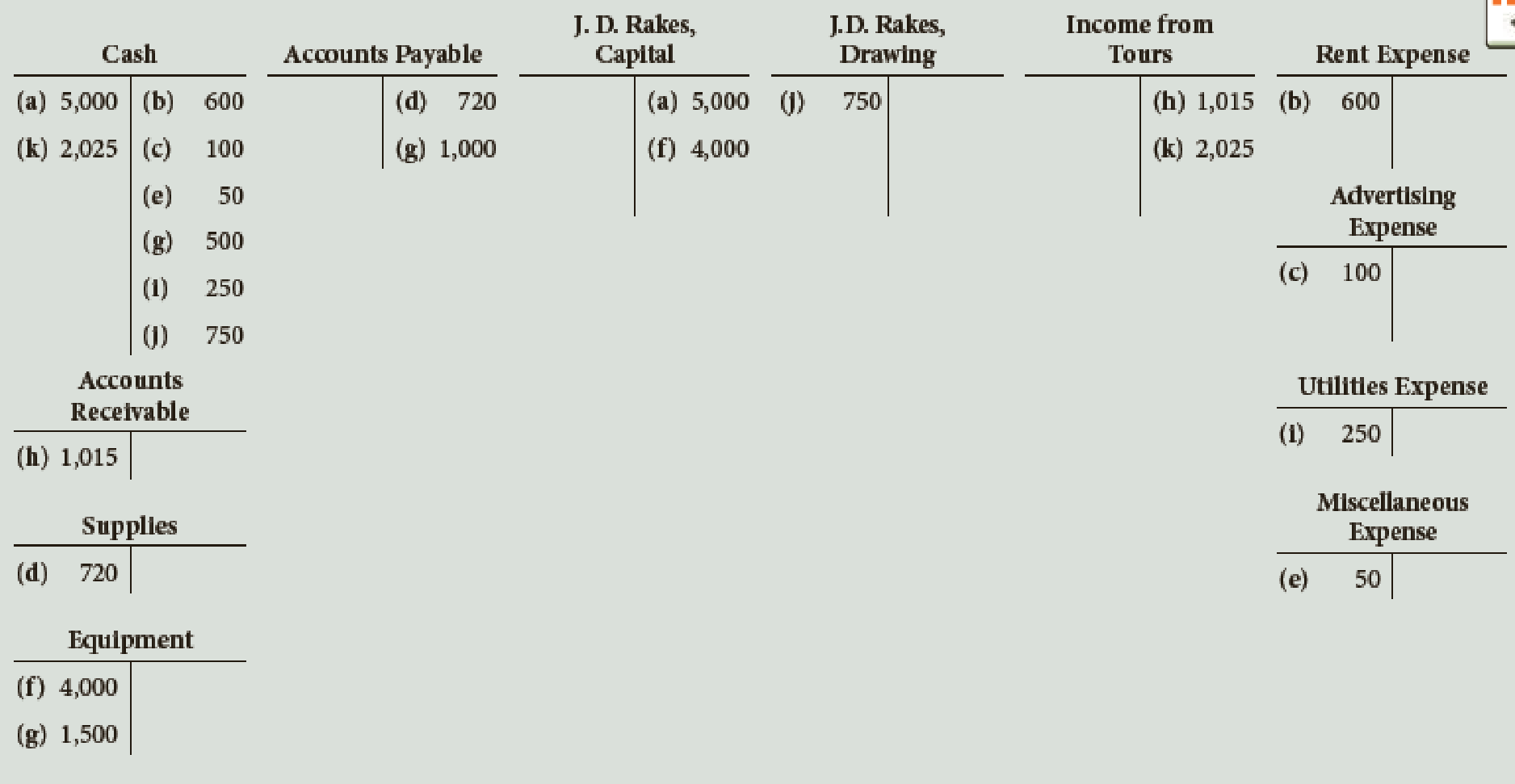Chapter 2, Problem 4E

Chapter
Section
Textbook Problem

During the first month of operation, Graham Expeditions recorded the following transactions. Describe what has happened in each of the transactions (a) through (k).To determine

Describe each of the transactions (a) through (k).

Explanation

Transaction analysis:

Transaction analysis is an economic event that causes impact on the value of the assets, liabilities or stockholder’s equity (increase or decrease) in an accounting equation.

Transaction (a):

Investment of \$5,000 is made by the owner in cash.

Transaction (b):

Rent is paid for the current month, \$600.

Transaction (c):

Transaction (d):

Supplies are bought on account for \$720.

Transaction (e):

Received and paid miscellaneous expense for \$50...

Still sussing out bartleby?

Check out a sample textbook solution.

See a sample solution

The Solution to Your Study Problems

Bartleby provides explanations to thousands of textbook problems written by our experts, many with advanced degrees!

Get Started